Search by Topic

Filter by: Content type:
Age range:
Challenge level:

There are 61 NRICH Mathematical resources connected to Angles - points, lines and parallel lines, you may find related items under Angles, Polygons, and Geometrical Proof.

Broad Topics > Angles, Polygons, and Geometrical Proof > Angles - points, lines and parallel linesRobotic Rotations

Age 11 to 16 Challenge Level:

How did the the rotation robot make these patterns?Olympic Turns

Age 7 to 11 Challenge Level:

This task looks at the different turns involved in different Olympic sports as a way of exploring the mathematics of turns and angles.Making Sixty

Age 14 to 16 Challenge Level:

Why does this fold create an angle of sixty degrees?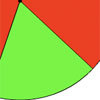Turning

Age 5 to 7 Challenge Level:

Use your mouse to move the red and green parts of this disc. Can you make images which show the turnings described?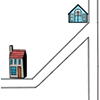Six Places to Visit

Age 7 to 11 Challenge Level:

Can you describe the journey to each of the six places on these maps? How would you turn at each junction?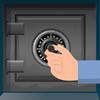How Safe Are You?

Age 7 to 11 Challenge Level:

How much do you have to turn these dials by in order to unlock the safes?Round and Round and Round

Age 11 to 14 Challenge Level:

Where will the point stop after it has turned through 30 000 degrees? I took out my calculator and typed 30 000 ÷ 360. How did this help?Semi-regular Tessellations

Age 11 to 16 Challenge Level:

Semi-regular tessellations combine two or more different regular polygons to fill the plane. Can you find all the semi-regular tessellations?Right Angles

Age 11 to 14 Challenge Level:

Can you make a right-angled triangle on this peg-board by joining up three points round the edge?Subtended Angles

Age 11 to 14 Challenge Level:

What is the relationship between the angle at the centre and the angles at the circumference, for angles which stand on the same arc? Can you prove it?Triangles in Circles

Age 11 to 14 Challenge Level:

Can you find triangles on a 9-point circle? Can you work out their angles?Octa-flower

Age 16 to 18 Challenge Level:

Join some regular octahedra, face touching face and one vertex of each meeting at a point. How many octahedra can you fit around this point?Estimating Angles

Age 7 to 14 Challenge Level:

How good are you at estimating angles?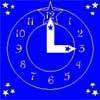Watch the Clock

Age 7 to 11 Challenge Level:

During the third hour after midnight the hands on a clock point in the same direction (so one hand is over the top of the other). At what time, to the nearest second, does this happen?Angle Trisection

Age 14 to 16 Challenge Level:

It is impossible to trisect an angle using only ruler and compasses but it can be done using a carpenter's square.Age 14 to 16 Challenge Level:

Join the midpoints of a quadrilateral to get a new quadrilateral. What is special about it?Age 16 to 18 Challenge Level:

A quadrilateral changes shape with the edge lengths constant. Show the scalar product of the diagonals is constant. If the diagonals are perpendicular in one position are they always perpendicular?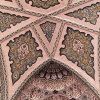Angles Inside

Age 11 to 14 Challenge Level:

Draw some angles inside a rectangle. What do you notice? Can you prove it?Shogi Shapes

Age 11 to 14 Challenge Level:

Shogi tiles can form interesting shapes and patterns... I wonder whether they fit together to make a ring?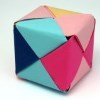Being Resilient - Primary Geometry

Age 5 to 11 Challenge Level:

Geometry problems at primary level that may require resilience.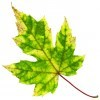Being Resourceful - Primary Geometry

Age 5 to 11 Challenge Level:

Geometry problems at primary level that require careful consideration.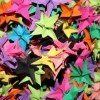Being Collaborative - Primary Geometry

Age 5 to 11 Challenge Level:

Geometry problems for primary learners to work on with others.Being Curious - Primary Geometry

Age 5 to 11 Challenge Level:

Geometry problems for inquiring primary learners.Polygon Rings

Age 11 to 14 Challenge Level:

Join pentagons together edge to edge. Will they form a ring?Same Length

Age 11 to 16 Challenge Level:

Construct two equilateral triangles on a straight line. There are two lengths that look the same - can you prove it?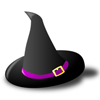Witch's Hat

Age 11 to 16 Challenge Level:

What shapes should Elly cut out to make a witch's hat? How can she make a taller hat?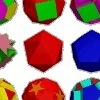Cylinder Cutting

Age 7 to 11 Challenge Level:

An activity for high-attaining learners which involves making a new cylinder from a cardboard tube.Which Solids Can We Make?

Age 11 to 14 Challenge Level:

Interior angles can help us to work out which polygons will tessellate. Can we use similar ideas to predict which polygons combine to create semi-regular solids?Interacting with the Geometry of the Circle

Age 5 to 16

Jennifer Piggott and Charlie Gilderdale describe a free interactive circular geoboard environment that can lead learners to pose mathematical questions.Dotty Circle

Age 7 to 11 Challenge Level:

Watch this film carefully. Can you find a general rule for explaining when the dot will be this same distance from the horizontal axis?Flight Path

Age 16 to 18 Challenge Level:

Use simple trigonometry to calculate the distance along the flight path from London to Sydney.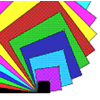Overlapping Squares

Age 7 to 11 Challenge Level:

Have a good look at these images. Can you describe what is happening? There are plenty more images like this on NRICH's Exploring Squares CD.Spirostars

Age 16 to 18 Challenge Level:

A spiropath is a sequence of connected line segments end to end taking different directions. The same spiropath is iterated. When does it cycle and when does it go on indefinitely?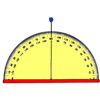Making Maths: Clinometer

Age 11 to 14 Challenge Level:

You can use a clinometer to measure the height of tall things that you can't possibly reach to the top of, Make a clinometer and use it to help you estimate the heights of tall objects.Making Maths: Equilateral Triangle Folding

Age 7 to 14 Challenge Level:

Make an equilateral triangle by folding paper and use it to make patterns of your own.Tessellating Hexagons

Age 11 to 14 Challenge Level:

Which hexagons tessellate?Orbiting Billiard Balls

Age 14 to 16 Challenge Level:

What angle is needed for a ball to do a circuit of the billiard table and then pass through its original position?Three Tears

Age 14 to 16 Challenge Level:

Construct this design using only compasses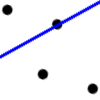Dotty Relationship

Age 7 to 11 Challenge Level:

Can you draw perpendicular lines without using a protractor? Investigate how this is possible.Age 14 to 16 Challenge Level:

Make five different quadrilaterals on a nine-point pegboard, without using the centre peg. Work out the angles in each quadrilateral you make. Now, what other relationships you can see?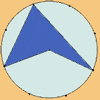Angle Measurement: an Opportunity for Equity

Age 11 to 16

Suggestions for worthwhile mathematical activity on the subject of angle measurement for all pupils.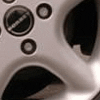Watch Those Wheels

Age 5 to 7 Challenge Level:

Have you ever noticed the patterns in car wheel trims? These questions will make you look at car wheels in a different way!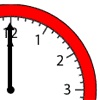Sweeping Hands

Age 7 to 11 Challenge Level:

Use your knowledge of angles to work out how many degrees the hour and minute hands of a clock travel through in different amounts of time.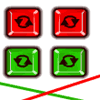Right Angle Challenge

Age 5 to 7 Challenge Level:

How many right angles can you make using two sticks?Pythagoras

Age 7 to 14

Pythagoras of Samos was a Greek philosopher who lived from about 580 BC to about 500 BC. Find out about the important developments he made in mathematics, astronomy, and the theory of music.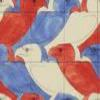Maurits Cornelius Escher

Age 7 to 14

Have you ever noticed how mathematical ideas are often used in patterns that we see all around us? This article describes the life of Escher who was a passionate believer that maths and art can be. . . .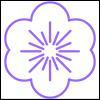Flower

Age 16 to 18 Challenge Level:

Six circles around a central circle make a flower. Watch the flower as you change the radii in this circle packing. Prove that with the given ratios of the radii the petals touch and fit perfectly.Lunar Angles

Age 16 to 18 Challenge Level:

What is the sum of the angles of a triangle whose sides are circular arcs on a flat surface? What if the triangle is on the surface of a sphere?LOGO Challenge 7 - More Stars and Squares

Age 11 to 16 Challenge Level:

Can you use LOGO to create a systematic reproduction of a basic design? An introduction to variables in a familiar setting.LOGO Challenge 8 - Rhombi

Age 7 to 16 Challenge Level:

Explore patterns based on a rhombus. How can you enlarge the pattern - or explode it?